### Select Language### AI社区ChatGPT注册使用圈 0个主题内容bug及解决办法圈 0个主题内容AI大学圈 2个主题内容# SOM 自组织映射神经网络

SOM是一种无监督的人工神经网络。不同于一般神经网络基于损失函数的反向传递来训练，它运用竞争学习(competitive learning)策略,依靠神经元之间互相竞争逐步优化网络。且使用近邻关系函数(neighborhood function)来维持输入空间的拓扑结构。

• 神经网络，竞争学习策略

• 无监督学习，不需要额外标签

• 非常适合高维数据的可视化，能够维持输入空间的拓扑结构

• 具有很高的泛化能力，它甚至能识别之前从没遇过的输入样本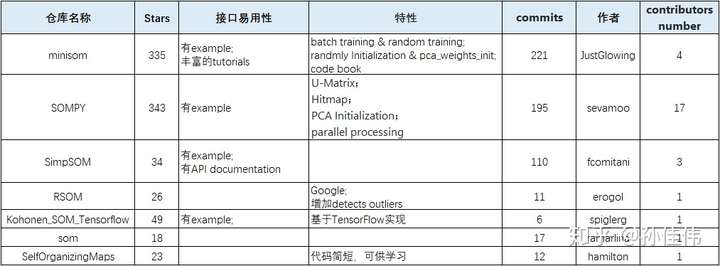## 网络结构结构

SOM的网络结构有2层：输入层、输出层(也叫竞争层)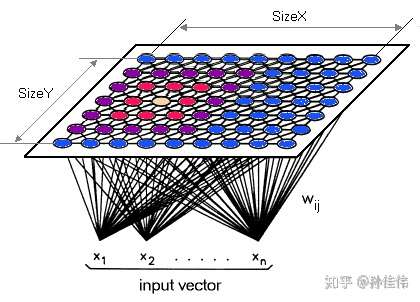SOM网络结构的区别主要在竞争层：可以有1维、2维(最常见的)

• Rectangular

• Hexagonal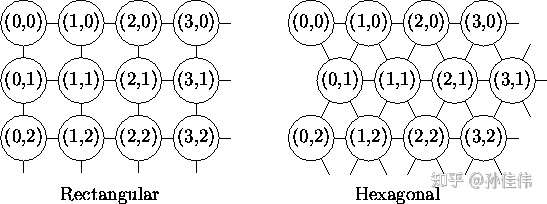N：训练样本的个数

## 训练计算过程

1. 遍历竞争层中每一个节点：计算Xi与节点之间的相似度(通常使用欧式距离)

2. 选取距离最小的节点作为优胜节点(winner node)，有的时也叫BMU(best matching unit)

W_v(s+1) = W_v(s) + θ(u,v,s) · α(s) · (D(t) - W_v(s))

θ(u,v,s)是对更新的约束，基于离BMU的距离  即neighborhood  function的返回值
W_v(s)是节点v当前的Wight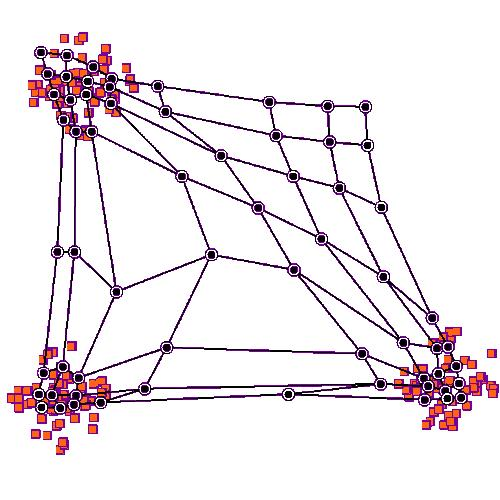## neighborhood function

neighborhood函数用来确定优胜节点对其近邻节点的影响强弱，即优胜邻域中每个节点的更新幅度。最常见的选择是高斯函数，它可以表征优胜邻域内，影响强弱与距离的关系。

```g = neighborhood_func(winner, sigma)
w_new = learning_rate * g * (x-w)```

winner是优胜节点在输出平面的坐标

sigma确定邻域范围，sig越大，邻域范围越大

sigma的取值范围：

• sigma必须大于0，否则没有神经元会被更新;

• 且sigma不能大于2维输出平面的边长

Bubble是函数很好地在计算成本与高斯核的准确性之间平衡。

## 优胜邻域内节点更新程度可视化：

```from numpy import outer, logical_and
size = 5
neigx = arange(size)
neigy = arange(size)```

## 高斯近邻函数：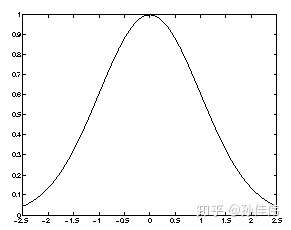```def gaussian(c, sigma):
"""Returns a Gaussian centered in c."""
d = 2*pi*sigma*sigma
ax = exp(-power(neigx-c, 2)/d)
ay = exp(-power(neigy-c, 2)/d)
return outer(ax, ay)  # the external product gives a matrix

out = gaussian((2,2),1)```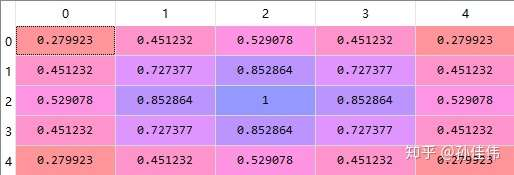sigma = 1

sigma其实是在控制这种随距离衰退的程度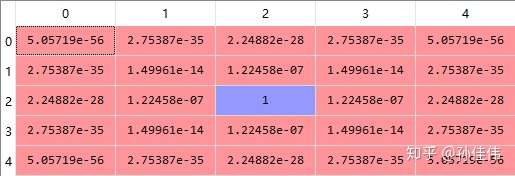sigma = 0.1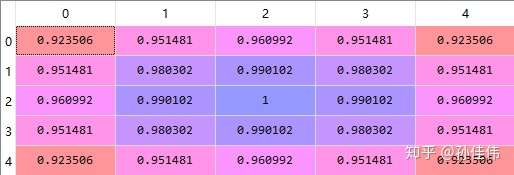sigma = 4

## Bubble近邻函数：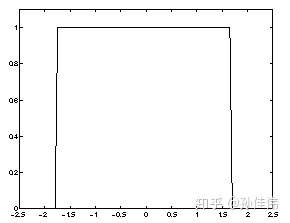Bubble函数：只要是在优胜邻域内的神经元，更新系数都是相同的

`(X > winner_x - sigma) & (X < winner_x + sigma)`

0.5：仅优胜节点

1.5：周围一圈

2.5：周围2圈

(1,2] 之间的取值，效果都一样  都是周围一圈
```def bubble( c, sigma):
"""Constant function centered in c with spread sigma.
sigma should be an odd value.
"""
ax = logical_and(neigx > c-sigma,
neigx < c+sigma)
ay = logical_and(neigy > c-sigma,
neigy < c+sigma)
return outer(ax, ay)*1.

out = bubble((2,2),sigma= 1.5)```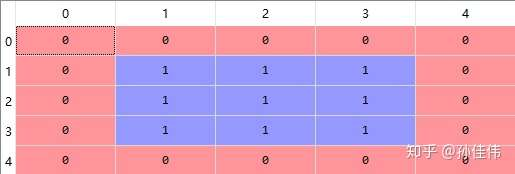sigma=1.5

`out = bubble((2,2),sigma= 0.5)`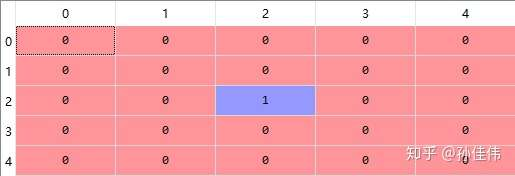sigma = 0.5

## 学习率α、邻域范围σ随时间衰减

SOM网络的另一个特点是，学习率和邻域范围随着迭代次数会逐渐衰减

## 衰减函数 decay func

sigma(t) = sigma / (1 + t/T)

learning_rate(t) = learning_rate / (1 + t/T)

t 代表当前迭代次数
T = 总迭代次数 / 2
`import numpy as npimport matplotlib.pyplot as pltnum_iteration = 1000T = num_iteration/2t = np.arange(0,num_iteration,10)decay_rate = 1 / (1+t/(T))plt.plot(decay_rate)`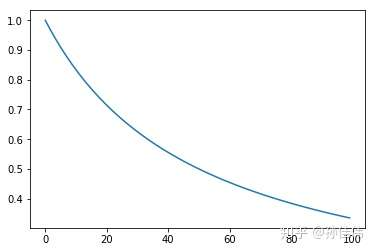## Initilization

• Random initialization：适用于对输入数据有很少或没有任何先验知识

• Initialization using initial samples：优点是初始时刻，网络节点就与输入数据的拓扑结构很相似

• Linear initialization(PCA)：让网络向输入数据能力最大的方向延伸

Principal component initialization is preferable (in dimension one) if the principal curve approximating the dataset can be univalently and linearly projected on the first principal component (quasilinear sets). For nonlinear datasets, however, random initiation performs better.

## 可视化

SOM本质是在逼近输入数据的概率密度，以下几种工具能非常好的可视化训练好的SOM网络

• U-Matrix

• Component Plane

## U-Matrix(unified distance matrix)

U-matrix包含每个节点与它的邻居节点(在输入空间)的欧式距离：

• 在矩阵中较小的值表示该节点与其邻近节点在输入空间靠得近

• 在矩阵中较大的值表示该节点与其邻近节点在输出空间离得远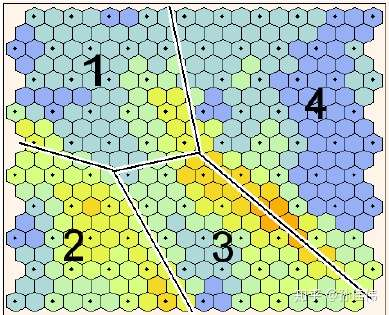彩色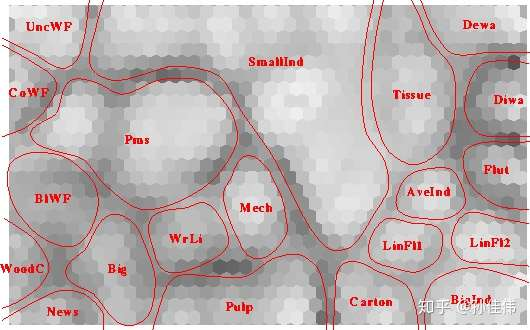黑白

```# miniSOM API
som.distance_map()```

## Component Plane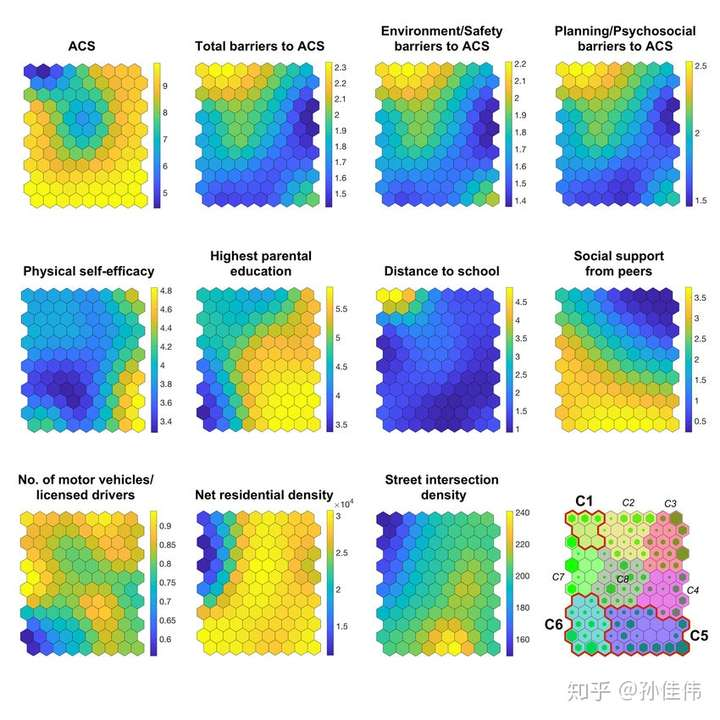Component plane可以理解成SOM网络的切片版本。每一个component plane包含了一个输入特征的相对分布。

## 解释性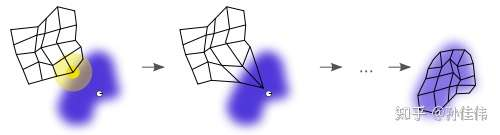逼近输入空间的过程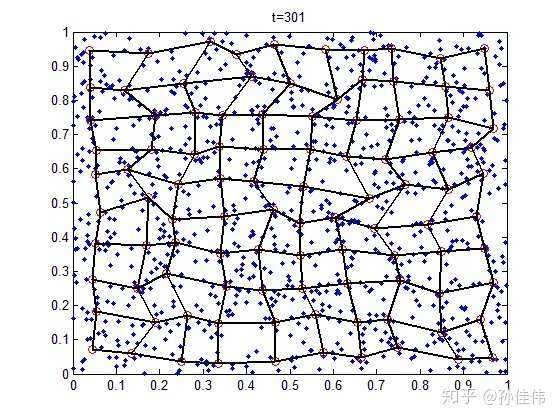## 总结：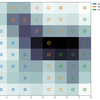## 附录：miniSomAPI参考

som.random_weights_init(X_train)：随机选取样本进行初始化 som.pca_weights_init(X_train)：PCA初始化

som.train_batch(X_train, max_iter, verbose=False)：逐个样本进行迭代 som.train_random(X_train, max_iter, verbose=False)：随机选样本进行迭代

• som.get_weights()：   Returns the weights of the neural network

• som.distance_map()：Returns the distance map of the weights

• som.activate(X)：         Returns the activation map to x   值越小的神经元，表示与输入样本 越匹配

• som.quantization(X)：Assigns a code book   给定一个 输入样本，找出该样本的优胜节点，然后返回该神经元的权值向量(每个元素对应一个输入单元)

• som.winner(X)：   给定一个 输入样本，找出该样本的优胜节点       格式：输出平面中的位置

• som.win_map(X)：将各个样本，映射到平面中对应的位置      返回一个dict   { position: samples_list }

• som.activation_response(X)：    返回输出平面中，各个神经元成为 winner的次数        格式为  1个二维矩阵

• quantization_error(量化误差)：  输入样本  与  对应的winner神经元的weight  之间的   平方根百科知识
2021-05-11 23:49:38加入圈子
• # 68条内容